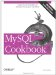# Summaries and NULL Values

7.9.1 Problem

You're summarizing a set of values that may include NULL values and you need to know how to interpret the results.

7.9.2 Solution

Understand how aggregate functions handle NULL values.

7.9.3 Discussion

Most aggregate functions ignore NULL values. Suppose you have a table expt that records experimental results for subjects who are to be given four tests each and that lists the test score as NULL for those tests that have not yet been administered:

```mysql> SELECT subject, test, score FROM expt ORDER BY subject, test;
+---------+------+-------+
| subject | test | score |
+---------+------+-------+
| Jane | A | 47 |
| Jane | B | 50 |
| Jane | C | NULL |
| Jane | D | NULL |
| Marvin | A | 52 |
| Marvin | B | 45 |
| Marvin | C | 53 |
| Marvin | D | NULL |
+---------+------+-------+```

By using a GROUP BY clause to arrange the rows by subject name, the number of tests taken by each subject, as well as the total, average, lowest, and highest score can be calculated like this,

```mysql> SELECT subject,
-> COUNT(score) AS n,
-> SUM(score) AS total,
-> AVG(score) AS average,
-> MIN(score) AS lowest,
-> MAX(score) AS highest
-> FROM expt GROUP BY subject;
+---------+---+-------+---------+--------+---------+
| subject | n | total | average | lowest | highest |
+---------+---+-------+---------+--------+---------+
| Jane | 2 | 97 | 48.5000 | 47 | 50 |
| Marvin | 3 | 150 | 50.0000 | 45 | 53 |
+---------+---+-------+---------+--------+---------+```

You can see from results in the column labeled n (number of tests) that the query counts only five values. Why? Because the values in that column correspond to the number of non-NULL test scores for each subject. The other summary columns display results that are calculated only from the non-NULL scores as well.

It makes a lot of sense for aggregate functions to ignore NULL values. If they followed the usual SQL arithmetic rules, adding NULL to any other value would produce a NULL result. That would make aggregate functions really difficult to use because you'd have to filter out NULL values yourself every time you performed a summary to avoid getting a NULL result. Ugh. By ignoring NULL values, aggregate functions become a lot more convenient.

However, be aware that even though aggregate functions may ignore NULL values, some of them can still produce NULL as a result. This happens if there's nothing to summarize. The following query is the same as the previous one, with one small difference. It selects only NULL test scores, so there's nothing for the aggregate functions to operate on:

```mysql> SELECT subject,
-> COUNT(score) AS n,
-> SUM(score) AS total,
-> AVG(score) AS average,
-> MIN(score) AS lowest,
-> MAX(score) AS highest
-> FROM expt WHERE score IS NULL GROUP BY subject;
+---------+---+-------+---------+--------+---------+
| subject | n | total | average | lowest | highest |
+---------+---+-------+---------+--------+---------+
| Jane | 0 | 0 | NULL | NULL | NULL |
| Marvin | 0 | 0 | NULL | NULL | NULL |
+---------+---+-------+---------+--------+---------+```

Even under these circumstances, the summary functions still return the most sensible value. The number of scores and total score per subject each are zero and are reported that way. AVG( ), on the other hand, returns NULL. An average is a ratio, calculated as a sum of values divided by the number of values. When there aren't any values to summarize, the ratio is 0/0, which is undefined. NULL is therefore the most reasonable result for AVG( ) to return. Similarly, MIN( ) and MAX( ) have nothing to work with, so they return NULL. If you don't want these functions to produce NULL in the query output, use IFNULL( ) to map their results appropriately:

```mysql> SELECT subject,
-> COUNT(score) AS n,
-> SUM(score) AS total,
-> IFNULL(AVG(score),0) AS average,
-> IFNULL(MIN(score),'Unknown') AS lowest,
-> IFNULL(MAX(score),'Unknown') AS highest
-> FROM expt WHERE score IS NULL GROUP BY subject;
+---------+---+-------+---------+---------+---------+
| subject | n | total | average | lowest | highest |
+---------+---+-------+---------+---------+---------+
| Jane | 0 | 0 | 0 | Unknown | Unknown |
| Marvin | 0 | 0 | 0 | Unknown | Unknown |
+---------+---+-------+---------+---------+---------+```

COUNT( ) is somewhat different with regard to NULL values than the other aggregate functions. Like other aggregate functions, COUNT(expr) counts only non-NULL values, but COUNT(*) counts rows, regardless of their content. You can see the difference between the forms of COUNT( ) like this:

```mysql> SELECT COUNT(*), COUNT(score) FROM expt;
+----------+--------------+
| COUNT(*) | COUNT(score) |
+----------+--------------+
| 8 | 5 |
+----------+--------------+```

This tells us that there are eight rows in the expt table but that only five of them have the score value filled in. The different forms of COUNT( ) can be very useful for counting missing values; just take the difference:

```mysql> SELECT COUNT(*) - COUNT(score) AS missing FROM expt;
+---------+
| missing |
+---------+
| 3 |
+---------+```

Missing and non-missing counts can be determined for subgroups as well. The following query does so for each subject. This provides a quick way to assess the extent to which the experiment has been completed:

```mysql> SELECT subject,
-> COUNT(*) AS total,
-> COUNT(score) AS 'non-missing',
-> COUNT(*) - COUNT(score) AS missing
-> FROM expt GROUP BY subject;
+---------+-------+-------------+---------+
| subject | total | non-missing | missing |
+---------+-------+-------------+---------+
| Jane | 4 | 2 | 2 |
| Marvin | 4 | 3 | 1 |
+---------+-------+-------------+---------+```MySQL Cookbook
ISBN: 059652708X
EAN: 2147483647
Year: 2005
Pages: 412
Authors: Paul DuBois